# 计算指数函数的和的对数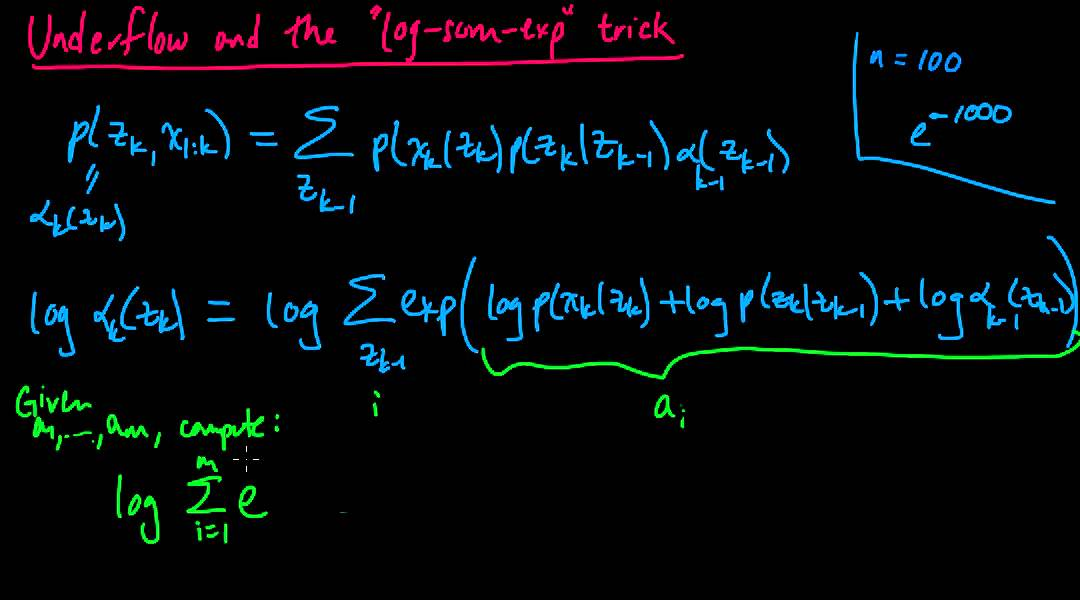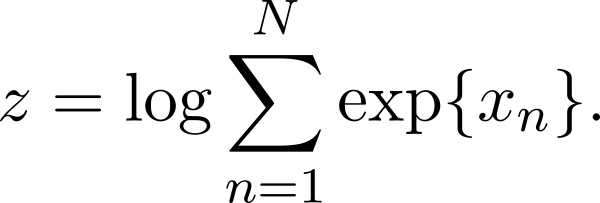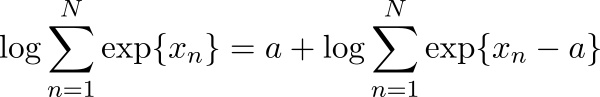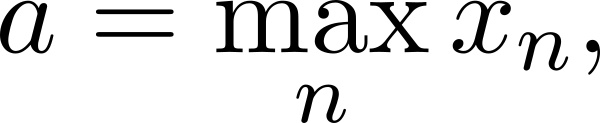## 证明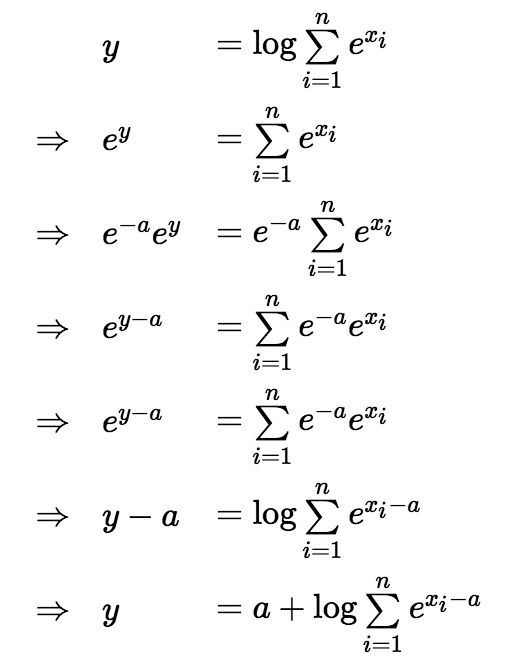## 实现

CRF++中的实现如下：

```#define MINUS_LOG_EPSILON  50
// log(exp(x) + exp(y));
//    this can be used recursivly
// e.g., log(exp(log(exp(x) + exp(y))) + exp(z)) =
// log(exp (x) + exp(y) + exp(z))
inline double logsumexp(double x, double y, bool flg)
{
if (flg)
return y;  // init mode
const double vmin = std::min(x, y);
const double vmax = std::max(x, y);
if (vmax > vmin + MINUS_LOG_EPSILON)
{
return vmax;
}
else
{
return vmax + std::log(std::exp(vmin - vmax) + 1.0);
}
}```

## Reference

https://hips.seas.harvard.edu/blog/2013/01/09/computing-log-sum-exp/

http://www.xarg.org/2016/06/the-log-sum-exp-trick-in-machine-learning/